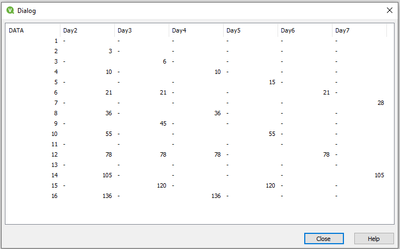# QlikView App Dev

Discussion Board for collaboration related to QlikView App Development.

Announcements
Action-Packed Learning Awaits! QlikWorld 2023. April 17 - 20 in Las Vegas: REGISTER NOW
cancel
Showing results for
Did you mean:Contributor

## Cumulative Sum on specific rows

Hi everyone,

this is my first post and I apologize if this has been asked before (actually, I couldn't find any proper solution) or if I'm missing to share some info.

Anyway, my problem is the following, I have a table with some data:

 DATA 1 2 3 4 5 6 7 8 9 10

and I would like to cumulate its values based on a "day" criteria. In other words, I'm trying to get this output:

 DATA 2 days 3 days 4 days 5 days 1 2 3 3 6 4 10 10 5 15 6 21 21 7 8 36 36 9 45 10 55 55

so, this means that I will need to cumulate starting always from the top of "DATA" (value=1) but skipping rows based on headers.

I hope my problem is clear

Thanks a lot for all your support!

Labels (2)

• ### QLIKVIEW Script

16 RepliesMVP

In the script or chart?Contributor
Author

It would be great to have it on script 🙂

Otherwise on the chart would be also ok.MVP

A crude script solution could be like:

``````Load
DATA,AccDATA,
If(Mod(RowNo(),2)=0, AccDATA) as Day2,
If(Mod(RowNo(),3)=0, AccDATA) as Day3,
If(Mod(RowNo(),4)=0, AccDATA) as Day4,
If(Mod(RowNo(),5)=0, AccDATA) as Day5,
If(Mod(RowNo(),6)=0, AccDATA) as Day6,
If(Mod(RowNo(),7)=0, AccDATA) as Day7 ;
DATA,
If(RowNo()=1,DATA, RangeSum(Peek('AccDATA'), DATA)) as AccDATA
;
DATA
1
2
3
4
5
6
7
8
9
10
11
12
13
14
15
16
];
Drop Field AccDATA;``````Contributor
Author

Thanks Tresesco, I tried but it works in part.

Indeed, if I add a Date column (to have a vision of what the situation would be each day) I end up with 7 times the same day for each day in my rangeContributor
Author

Thanks to the community for the support!

I will close this topic since there are no more proposals.  I will work on Tresesco solution and find a way!

Thanks!Specialist II

One more way to do this in frontend is to create 4 expression like the below

Expression:

2nd day  =  sum({<DATA={'2','4','6','8','10'}>} aggr( RangeSum(above(sum(DATA),0,RowNo())),DATA))

3rd day  =  sum({<DATA={'3','6','9'}>} aggr( RangeSum(above(sum(DATA),0,RowNo())),DATA))

4th day  =  sum({<DATA={'4','8'}>} aggr( RangeSum(above(sum(DATA),0,RowNo())),DATA))

5th day  =  sum({<DATA={'5','10'}>} aggr( RangeSum(above(sum(DATA),0,RowNo())),DATA))Contributor
Author

Hi Qliksus,

thanks for your reply! This could be interesting but I have tons of rows..Community Browser